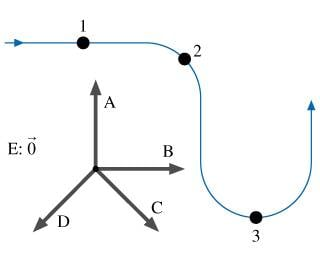# Ridiculously simple acceleration and velocity vectors, but I can't get them

Ridiculously simple acceleration and velocity vectors, but I can't get them....

We're supposed to answer a series of questions based on this diagram, but I am so confused by the answers.

## Homework Statement

A car travels at constant speed along the curved path shown in the figure below. Five possible vectors are also shown in the figure; the letter E represents the zero vector.These are the 3 questions (along with the correct answers from the key):

1. Which vector best represents the car's acceleration at point 1?

2. Which vector best represents the car's acceleration at point 2?

3. Which vector best represents the car's acceleration at point 3?

I don't get these answers at all, first the question tells me the car is traveling at a constant speed, (which I answered in question 1, E) Then it says it's D for question 2, then it's A for question 3? I don't get how these vectors connect together at all, and it's completely stumped me.

Thanks.